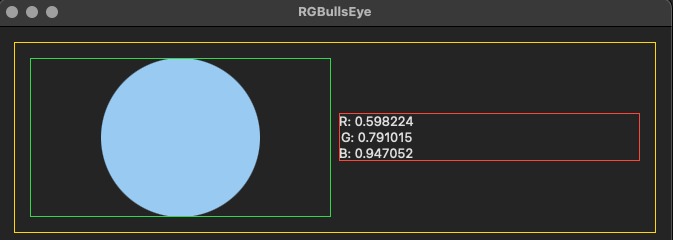# Questions about RGBBullsEye on macOS

I reference your code and running on macOS.

Q1)How do I force divide content view width in half?
Q2)How do I place the circle on the rightmost in green area?
Q3)How do I avoid a gap between green area and red area?

thx``````struct CircleRGB: View {
@Binding var rgb: RGB
var body: some View {
HStack {
Circle()
.fill(Color(rgb: self.rgb))
.frame(maxWidth: .infinity, alignment: .trailing)
.border(.green)

VStack {
Text("R: \(self.rgb.red)")
Text("G: \(self.rgb.green)")
Text("B: \(self.rgb.blue)")
}
.border(.red)
}
.border(.yellow)
}
}
``````

hi diuming,

1. use `GeometryReader` (chapter 13)
2. use `Spacer()` (chapter 7)
3. use `HStack(spacing: 0)`. You can also use a negative spacing value

Hi Audrey,

1. I will try it soon.
2. It’s no effect.
3. It works very well.

``````    var body: some View {
HStack(spacing: 0) {
HStack {
Spacer()
Circle()
.fill(Color(rgb: self.rgb))
.frame(maxWidth: .infinity, alignment: .trailing)
}
.border(.green)

VStack {
Text("R: \(self.rgb.red)")
Text("G: \(self.rgb.green)")
Text("B: \(self.rgb.blue)")
}
.border(.red)
}
.border(.yellow)
}
``````

strange that Spacer() doesn’t work. Try this:

``````HStack {
HStack {
Rectangle()
.fill(Color(.clear))
Circle()
.fill(Color(rgbStruct: self.rgb))
}
.frame(maxWidth: .infinity)
.border(.green)
// ...
``````

The `circle` is moved to right, but not `rightmost`.
thanks.